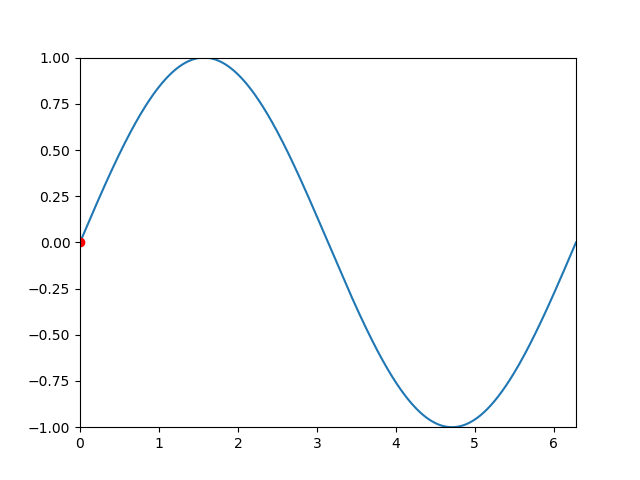# Matplotlib 调用ImageMagick输出gif文件

ImageMagick是免费软件，可以即用型二进制分发形式提供，也可以作为源代码提供，您可以在开放应用程序和专有应用程序中使用，复制，修改和分发它们。它是在派生的Apache 2.0 许可下分发的。

ImageMagick利用多个计算线程来提高性能，并且可以读取，处理或写入兆，千兆或兆像素图像大小。

ImageMagick的官网有：

https://imagemagick.org/index.php

http://www.imagemagick.com.cn/ImageMagick-7.0.10-53-Q16-HDRI-x64-dll.exeplt.rcParams['animation.convert_path'] = r'D:\ImageMagick\magick.exe'

writer = animation.ImageMagickFileWriter(fps = 30)
anim.save('ani-057.gif', writer=writer)


class matplotlib.animation.ImageMagickWriter(fps=5, codec=None, bitrate=None, extra_args=None, metadata=None)

• fps：表示帧速率, 默认值: 5

• codec：表示使用编码器，可以是字符串或None, 默认: rcParams[“animation.codec”] (default: ‘h264’)

• bitrate：表示位速率，kb/s，速率越高视频质量越好，但体积越来越大, 默认值: rcParams[“animation.bitrate”] (default: -1)

• extra_args：表示命名行参数列表，可选。import numpy as np
from matplotlib import pyplot as plt
from matplotlib import animation

plt.rcParams['animation.convert_path'] = r'D:\ImageMagick\magick.exe'

#
TWOPI = 2*np.pi
#
fig, ax = plt.subplots()

t = np.arange(0.0, TWOPI, 0.001)
s = np.sin(t)
l = plt.plot(t, s)

ax = plt.axis([0,TWOPI,-1,1])
#初始化
redDot, = plt.plot(, [np.sin(0)], 'ro')

#动画回调函数
def animate(i):
redDot.set_data(i, np.sin(i))
return redDot,

# 使用animate函数
anim = animation.FuncAnimation(fig, animate, frames=np.arange(0.0, TWOPI, 0.1),
interval=10, blit=True, repeat=False)

#
writer = animation.ImageMagickFileWriter(fps = 30)
anim.save('ani-057.gif', writer=writer)

plt.show()


• 回顶